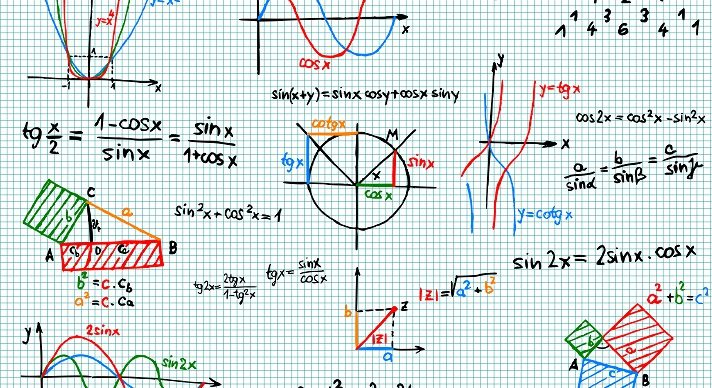# Integrated Mathematics III### Course Overview

Acellus Integrated Mathematics III, taught by Patrick Mara, is the last course of a three-part series that includes algebra, geometry, probability, and statistics. This high school math pathway is patterned after an approach typically seen internationally. Acellus Integrated Mathematics III is A-G Approved through the University of California.This course was developed by the International Academy of Science. Learn More

### Scope and Sequence

Unit 1 In this introductory unit, students will be introduced to sets of numbers, simplifying and evaluating, and solving equations. They will also learn about solving inequalities and absolute value. Unit 2 In this unit students will delve into the definition of a function, direct variation, slope-intercept, point-slope, piecewise defined functions, linear models, and transformations of functions. Students will also study absolute value functions and inequalities in two variables. Unit 3 In this unit students will learn to solve graphically, use substitution, elimination, systems of inequalities, and linear programming. They will also learn systems in three variables, and matrices. Unit 4 In this unit students will learn to include using transformations.  They will also study standard form, quadratic regression, factoring, quadratic equations, completing the square, and the quadratic formula. Students will also learn how to use quadratic inequalities, complex numbers, and representing operations of complex numbers. Unit 5 In this unit students will be introduced to polynomial functions, factored form, polynomial equations, dividing polynomials, synthetic division, and finding roots. Students will also study the fundamental theorem of algebra, graphing polynomial functions, the binomial theorem, and regression. Unit 6 In this unit students will explore properties of exponents, rational exponents, adding radicals, and solving radical equations. Additionally students will learn about the composition of functions, inverse functions, and graphing radical functions. Unit 7 Students begin this unit by learning how to use exponential functions, logarithmic functions, and properties of logarithms. Additionally, students will learn how to solve exponential and logarithmic equations, and how to find compound interest. Unit 8 In this unit students will study inverse variation, reciprocal functions, graphs of rational functions, multiplication and division of rational expressions, and adding and subtracting rational expressions. They will learn about solving rational equations, solving rational inequalities, and number theory. Unit 9 Within this unit students will learn about explicit versus recursive definitions, arithmetic sequences, and geometric sequences. They will also explore arithmetic series and geometric series. Unit 10 In this unit students will learn about permutations and combinations, probability, theoretical and experimental probability, multiple events, conditional probability, probability models, and analyzing data. Students will also learn about standard deviation, how to use samples and surveys, binomial distributions, normal distributions, probability distribution functions, and expected value. Unit 11 In this unit students will gain knowledge of the unit circle, radian measure, the sine function, the cosine function, and the tangent function. Students will also gain understanding of translating trigonometric functions, and reciprocal trigonometric functions. Unit 12 In this unit students will study basic identities, solving trig equations, inverse trig functions, and right triangles. They will explore the law of sines and the law of cosines. Unit 13 In this unit students will learn about areas of parallelograms, triangles, trapezoids, rhombuses, and kites. Students will also learn about areas of regular polygons, and perimeters and areas of similar figures, as well as trigonometry and area. Unit 14 In this concluding unit students will learn about polyhedra. They will study surface area and volume of prisms, cylinders, pyramids, and cones. Students will conclude with learning about surface areas and volumes of spheres, and areas and volumes of similar solids.